# Video Tutorial 5 SQL. Joins; inner join, left join, right join with Mysql Workbench

SQL joins are used to combine rows from two or more tables.

## SQL JOIN

An SQL JOIN clause is used to combine rows from two or more tables, based on a common field between them.

The most common type of join is: SQL INNER JOIN (simple join). An SQL INNER JOIN return all rows from multiple tables where the join condition is met.

#### INNER JOIN SYNTAX

```SELECT column_name(s)
FROM table1
INNER JOIN table2
ON table1.column_name=table2.column_name;```

or

```SELECT column_name(s)
FROM table1
JOIN table2
ON table1.column_name=table2.column_name;```

Notice that INNER JOIN is the same as JOIN.#### INNER JOIN Example

In our example we are combining all the rows of the tables "publisher" and "books" where the condition books.idpublisher=publisher.idpublisher is met.

##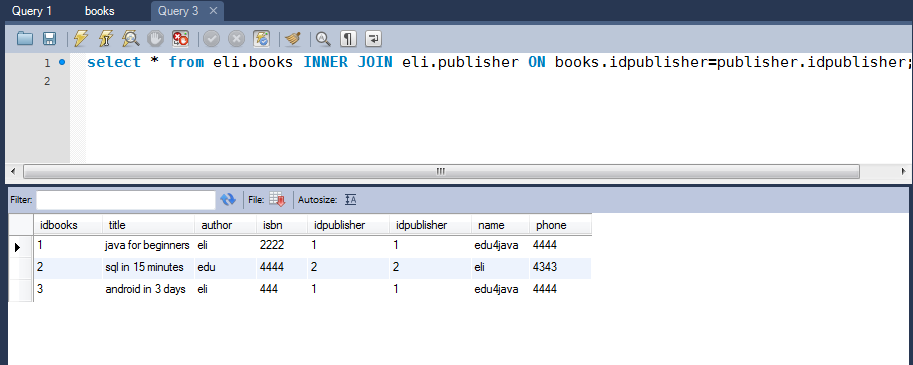SQL LEFT JOIN Keyword

The LEFT JOIN keyword returns all rows from the left table (table1), with the matching rows in the right table (table2). The result is NULL in the right side when there is no match.

#### SQL LEFT JOIN Syntax

``` SELECT column_name(s)
FROM table1
LEFT JOIN table2
ON table1.column_name=table2.column_name;
```

in some databases LEFT JOIN is called LEFT OUTER JOIN;

``` SELECT column_name(s)
FROM table1
LEFT OUTER JOIN table2
ON table1.column_name=table2.column_name;```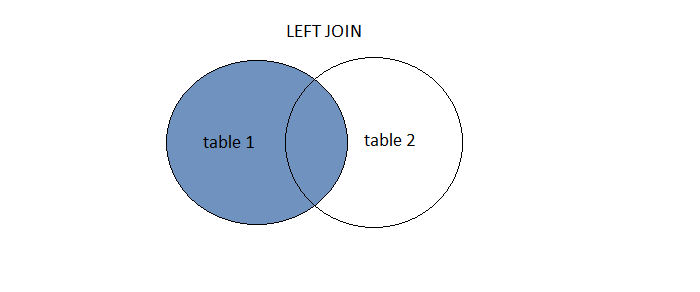#### SQL LEFT JOIN Example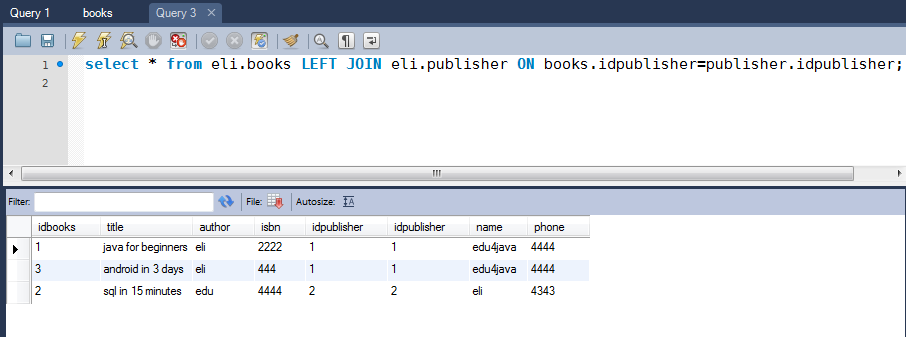Here we can see the same result as in INNER JOIN but this is because all the registers of "books" have an "idpublisher" matching the "idpublisher" of the table "publisher". If in "books" we write a new register and we write an "idpublisher" which doesn´t have a match with any "idpublisher" in the table "publisher" (idpublisher=0);When we write our left join query we are not going to have the registers of the table "publisher" in our result set;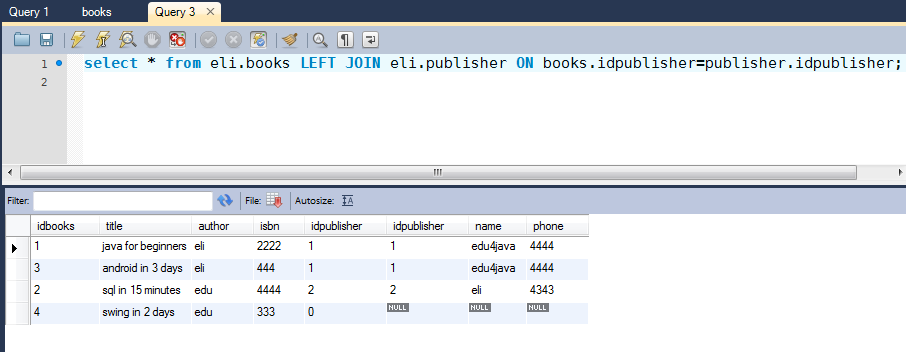## SQL RIGHT JOIN Keyword

The RIGHT JOIN keyword returns all rows from the right table (table2), with the matching rows in the left table (table1). The result is NULL in the left side when there is no match.

#### SQL RIGHT JOIN Syntax

```  SELECT column_name(s)
FROM table1
RIGHT JOIN table2
ON table1.column_name=table2.column_name;
```

or

```  SELECT column_name(s)
FROM table1
RIGHT OUTER JOIN table2
ON table1.column_name=table2.column_name;
```#### SQL RIGHT JOIN Example

We can change the order of the tables in the query so that we can see that it is the same that in LEFT JOIN but the other way round;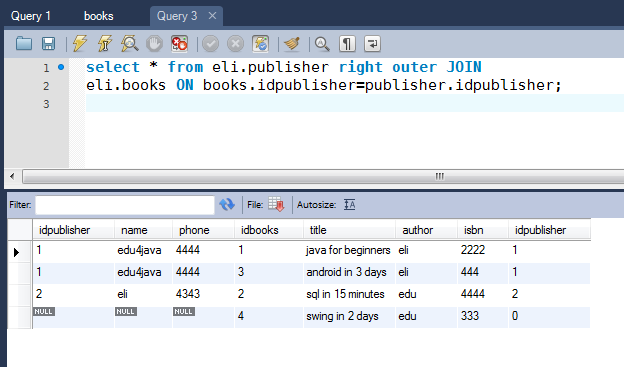<< Previous Next >>